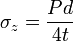Pressure Vessel , Thin Wall Hoop and Longitudinal Stresses

For the thin-walled assumption to be valid the vessel must have a wall thickness of no more than about one-tenth (often cited as one twentieth) of its radius. The classic equation for hoop stress created by an internal pressure on a thin wall cylindrical pressure vessel is:

σθ = PDm /2t for the Hoop Stress
Thin Wall Pressure Vessel Hoop Stress Calculator

where:

• P = is the internal pressure
• t = is the wall thickness
• r = is the inside radius of the cylinder.
• Dm = Mean Diameter (Outside diameter - t). Mean diameter of OD and ID...
• σθ = is the hoop stress.The hoop stress equation for thin shells is also approximately valid for spherical vessels, including plant cells and bacteria in which the internal turgor pressure may reach several atmospheres.

Inch-pound-second system (IPS) units for P are pounds-force per square inch (psi). Units for t, and d are inches (in). SI units for P are pascals (Pa), while t and d=2r are in meters (m).

Longitudinal Stress Thin Walled Pressure Vessel:

When the vessel has closed ends the internal pressure acts on them to develop a force along the axis of the cylinder. This is known as the axial or longitudinal stress and is usually less than the hoop stress.$Equation$

Though this may be approximated to$Equation$
Thin Wall Pressure Vessel Longitudinal Stress Calculator

Where:

P = Pressure
d = Mean Diameter (Outside diameter - t). Mean diameter of OD and ID...
t = Wall Thickness= Logitudinal Stress© Copyright 2000 - 2019, by Engineers Edge, LLC www.engineersedge.comMembership Register | LoginHomeEngineering Book StoreEngineering ForumExcel App. DownloadsOnline Books & ManualsEngineering NewsEngineering VideosEngineering CalculatorsEngineering ToolboxGD&T Training Geometric Dimensioning TolerancingDFM DFA TrainingTraining Online EngineeringAdvertising CenterCopyright Notice